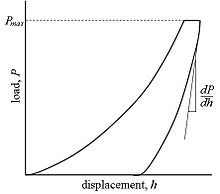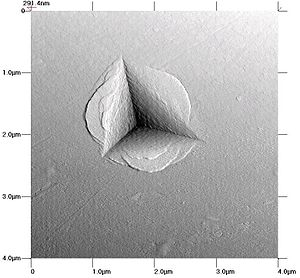# Nanoindentation

﻿
Nanoindentation

Nanoindentation is a variety of indentation hardness tests applied to small volumes. Indentation is perhaps the most commonly applied means of testing the mechanical properties of materials. The nanoindentation technique was developed in the mid-1970s to measure the hardness of small volumes of material.[citation needed]

## Background

In a traditional indentation test (macro or micro indentation), a hard tip whose mechanical properties are known (frequently made of a very hard material like diamond) is pressed into a sample whose properties are unknown. The load placed on the indenter tip is increased as the tip penetrates further into the specimen and soon reaches a user-defined value. At this point, the load may be held constant for a period or removed. The area of the residual indentation in the sample is measured and the hardness, H, is defined as the maximum load, Pmax, divided by the residual indentation area, Ar, or$H=\frac{P_{max}} {A_{r}}$.

For most techniques, the projected area may be measured directly using light microscopy. As can be seen from this equation, a given load will make a smaller indent in a "hard" material than a "soft" one.

This technique is limited due to large and varied tip shapes, with indenter rigs which do not have very good spatial resolution (the location of the area to be indented is very hard to specify accurately). Comparison across experiments, typically done in different laboratories, is difficult and often meaningless. Nanoindentation improves on these macro and micro indentation tests by indenting on the nanoscale with a very precise tip shape, high spatial resolutions to place the indents, and by providing real-time load-displacement (into the surface) data while the indentation is in progress.

## NanoindentationFigure 1. Schematic of load-displacement curve for an instrumented nanoindentation test

In nanoindentation small loads and tip sizes are used, so the indentation area may only be a few square micrometres or even nanometres. This presents problems in determining the hardness, as the contact area is not easily found. Atomic force microscopy or scanning electron microscopy techniques may be utilized to image the indentation, but can be quite cumbersome. Instead, an indenter with a geometry known to high precision (usually a Berkovich tip, which has a three-sided pyramid geometry) is employed. During the course of the instrumented indentation process, a record of the depth of penetration is made, and then the area of the indent is determined using the known geometry of the indentation tip. While indenting various parameters, such as load and depth of penetration, can be measured. A record of these values can be plotted on a graph to create a load-displacement curve (such as the one shown in Figure 1). These curves can be used to extract mechanical properties of the material.

• Young's Modulus: The slope of the curve, dP / dh, upon unloading is indicative of the stiffness S of the contact. This value generally includes a contribution from both the material being tested and the response of the test device itself. The stiffness of the contact can be used to calculate the reduced Young's modulus Er:$E_r=\frac{1}{\beta}\frac{\sqrt{\pi}}{2}\frac{S}{\sqrt{A_p(h_c)}},$

where Ap(hc) is the projected area of the indentation at the contact depth hc , and β is a geometrical constant on the order of unity. Ap(hc) is often approximated by a fitting polynomial as shown below for a Berkovich tip:$A_p(h_c)=C_0h_c^2+C_1h_c^1+C_2h_c^{1/2}+C_3h_c^{1/4}+\ldots+C_8h_c^{1/128}$

Where C0 for a Berkovich tip is 24.5 while for a cube corner (90°) tip is 2.598. The reduced modulus Er is related to Young's modulus Es of the test specimen through the following relationship from contact mechanics:$1/E_r=(1-\nu_i^2)/E_i+(1-\nu_s^2)/E_s.$

Here, the subscript i indicates a property of the indenter material and ν is Poisson's ratio. For a diamond indenter tip, Ei is 1140 GPa and νi is 0.07. Poisson’s ratio of the specimen, νs, generally varies between 0 and 0.5 for most materials (though it can be negative) and is typically around 0.3.An AFM image of an indent left by a Berkovich tip in a Zr-Cu-Al metallic glass; the plastic flow of the material around the indenter is apparent.
• Hardness: There are two different types of hardness that can be obtained from a nano indenter: one is as in traditional macroindentation tests where one attains a single hardness value per experiment; the other is based on the hardness as the material is being indented resulting in hardness as a function of depth.$H=\frac{P_{max}} {A_{r}}.$

The hardness is given by the equation above, relating the maximum load to the indentation area. The area can be measured after the indentation by in-situ atomic force microscopy, or by 'after-the event' optical (or electron) microscopy. An example indentation image, from which the area may be determined, is shown at right.

Some nanoindenters use an area function based on the geometry of the tip, compensating for elastic load during the test. Use of this area function provides a method of gaining real-time nanohardness values from a load-displacement graph. However, there is some controversy over the use of area functions to estimate the residual areas versus direct measurement.[citation needed] An area function Ap(hc) typically describes the projected area of an indent as a 2nd-order polynomial function of the indenter depth h. Exclusive application of an area function in the absence of adequate knowledge of material response can lead to misinterpretation of resulting data. Cross-checking of areas microscopically is to be encouraged.

• Strain-rate sensitivity: The strain-rate sensitivity of the flow stress m is defined as$m=\frac{\partial\ln{\sigma}}{\partial\ln{\dot{\varepsilon}}},$

where$\sigma=\sigma(\dot{\varepsilon})$ is the flow stress and$\dot{\varepsilon}$ is the strain rate produced under the indenter. For nanoindentation experiments which include a holding period at constant load (i.e. the flat, top area of the load-displacement curve), m can be determined from$d\ln{H}=m d\ln{\dot{\varepsilon_p}}+n d\ln{h_p}.$

The subscripts p indicate these values are to be determined from the plastic components only.

• Activation volume: Interpreted loosely as the volume swept out by dislocations during thermal activation, the activation volume V * is$V^*=9 k_B T \frac{\partial\ln{\dot{\varepsilon}}}{\partial H},$

where T is the temperature and kB is Boltzmann's constant. From the definition of m, it is easy to see that$V^*\propto (H m)^{-1}$.

## Software

Software is the best method to analyze the nanoindentation load versus displacement curves for hardness and elastic modulus calculations. The Martens hardness, HM, is relatively simple software for a novice programmer to develop. The software starts by searching for the maximum displacement, hmax, point and maximum load, Pmax.$HM=\frac{P_{max}} {A_{s}}.$

The displacement is used to calculate the contact surface area, As, based on the indenter geometry. For a perfect Berkovich indenter the relationship is$A_s = 24.5 h_{max}^2$.

The Indentation hardness, HIT is defined slightly different.$H_{IT}=\frac{P_{max}} {A_{p}}.$

Here, the hardness is related to the projected contact area Ap.

As the indent size decreases the error caused by tip rounding increases. The tip wear can be accounted for within the software by using a simple polynomial function. As the indenter tip wears the C1 value will increase. The user will can enter the values for C0 and C1 based on direct measurements such as SEM or AFM images of the indenter tip or indirectly by using a material of known elastic modulus or an AFM image of the an indentation.$A_p = C_0 h_{max}^2 + C_1 h_{max}.$$P = k \left(h-h_{f}\right)^m.$

## Devices

The construction of a depth-sensing indentation system is made possible by the inclusion of very sensitive displacement and load sensing systems. Load transducers must be capable of measuring forces in the micronewton range and displacement sensors are very frequently capable of sub-nanometer resolution. Environmental isolation is crucial to the operation of the instrument. Vibrations transmitted to the device, fluctuations in atmospheric temperature and pressure, and thermal fluctuations of the components during the course of an experiment can cause significant errors.

The ability to conduct nanoindentation studies with nanometer depth, and sub-nanonewton force resolution is also possible using a standard AFM setup. The AFM allows for nanomechanical studies to be conducted alongside topographic analyses, without the use of dedicated instruments. Load-displacement curves can be collected similarly for a variety of materials, and mechanical properties can be directly calculated from these curves.

## Limitations

Conventional nanoindentation methods for calculation of Modulus of elasticity (based on the unloading curve) are limited to linear, isotropic materials. Problems associated with the "pile-up" or "sink-in" of the material on the edges of the indent during the indentation process remain a problem that is still under investigation.

It is possible to measure the pile-up contact area using computerized image analysis of atomic force microscope (AFM) images of the indentations. This process also depends on the linear isotropic elastic recovery for the indent reconstruction.

Wikimedia Foundation. 2010.

### Look at other dictionaries:

• nanoindentation — noun An indentation test carried out at nanoscale, such as by use of a nanoindenter See Also: nanoindenter …   Wiktionary

• Nanoindenter — A nanoindenter A nanoindenter is the main component for indentation hardness tests used in nanoindentation. Since the mid 1970’s nanoindentation has become the primary method for measuring and testing very small volumes of mechanical properties.… …   Wikipedia

• Indentation hardness — tests are used to determine the hardness of a material to deformation. Several such tests exist, wherein the examined material is indented until an impression is formed; these tests can be performed on a macroscopic or microscopic scale. When… …   Wikipedia

• Diamond-like carbon — A ta C thin film on silicon (15 mm diameter) exhibiting regions of 40 nm and 80 nm thickness …   Wikipedia

• Mineralized tissues — Mineralized tissues: sea sponge, sea shells, conch, dentin, radiolarian, antler, bone Mineralized tissues are biological tissues that incorporate minerals into soft matrices. Typically these tissues form a protective shield or structural… …   Wikipedia

• Nanoindentierung — Die Nanoindentierung (auch instrumentierter Eindringversuch) ist eine Methode der Werkstoffprüfung zur Bestimmung der Härte von Materialien auf kleinen Längenskalen (Nanometer, nm). Hauptanwendungsgebiet ist die Härtebestimmung an dünnen… …   Deutsch Wikipedia

• Carbon nanotube — Not to be confused with Carbon fiber. Part of a series of articles on Nanomaterials Fullerenes …   Wikipedia

• Collagen — Tropocollagen triple helix Collagen /ˈkɒlədʒɨn/ is a group of naturally occurring proteins found in animals, especially in the flesh and connective tissues of mammals. It is the main component of connectiv …   Wikipedia

• Heinrich Hertz — Infobox Scientist name = Heinrich Rudolf Hertz image width = 230px birth date = birth date|1857|2|22|mf=y birth place = Hamburg, Germany residence = Germany nationality = German death date = death date and age|1894|1|1|1857|2|22|mf=y death place …   Wikipedia

• Mechanosynthesis — Part of a series of articles on Molecular Nanotechnology …   Wikipedia